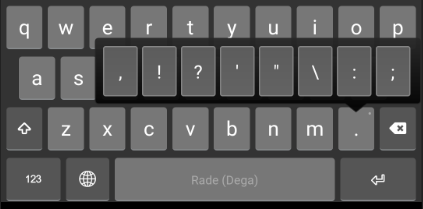### Keyman.com Homepage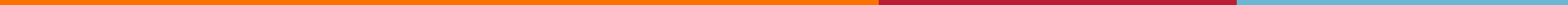Keyman.com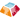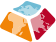## Dega Keyboard

Dega keyboard is created for indigenous people in the Central Highlands of Vietnam, to preserve their writing, this Dega keyboard can be used by Êđê, Jơrai, Kơ’Ho, Mơnông, Bahnar and many other indigenous ethnic groups.

For how to type certain characters other than the usual Latin characters, please refer to the following bullet points.

## Upper Case

• E + E = Ê
• E + X = Ĕ
• E + E + X = Ê̆
• U + U = Ư
• U + X = Ŭ
• U + U + X = Ư̆
• U + W = Ư
• U + W + X = Ư̆
• I + X = Ĭ
• Y + X = Y̆
• O + O = Ô
• O + X = Ŏ
• O + O + X = Ô̆
• O + W = Ơ
• O + W + X = Ơ̆
• A + A = Â
• A + W = Ă
• A + X = Ă
• A + A + X = Â̆
• C + C = Č
• B + B = Ƀ
• N + N = Ñ
• D + D = Đ

## Lower Case

• e + e = ê
• e + x = ĕ
• e + e + x = ê̆
• u + u = ư
• u + x = ŭ
• u + u + x = ư̆
• u + w = ư
• u + w + x = ư̆
• i + x = ĭ
• y + x = y̆
• o + o = ô
• o + x = ŏ
• o + o + x = ô̆
• o + w = ơ
• o + w + x = ơ̆
• a + a = â
• a + w = ă
• a + x = ă
• a + a + x = â̆
• c + c = č
• b + b = ƀ
• n + n = ñ
• d + d = đ

# Keyboard Layout

## Phone

### Longpress

Keys with a little dot on the top right can be pressed and held for more keys.